Question
Simplify each expression. Thanks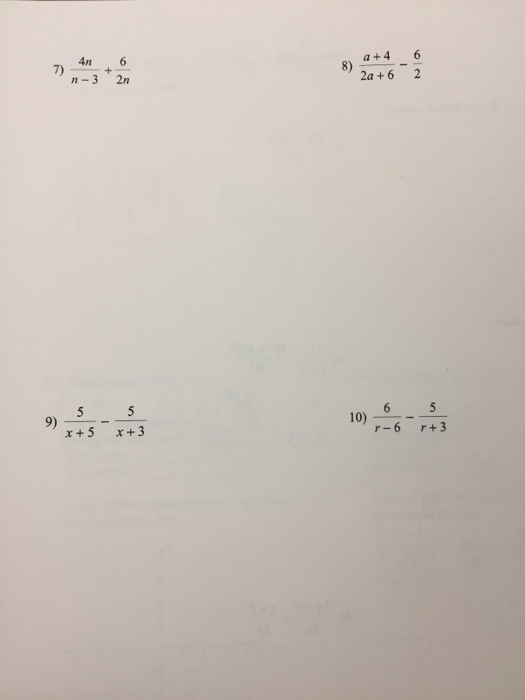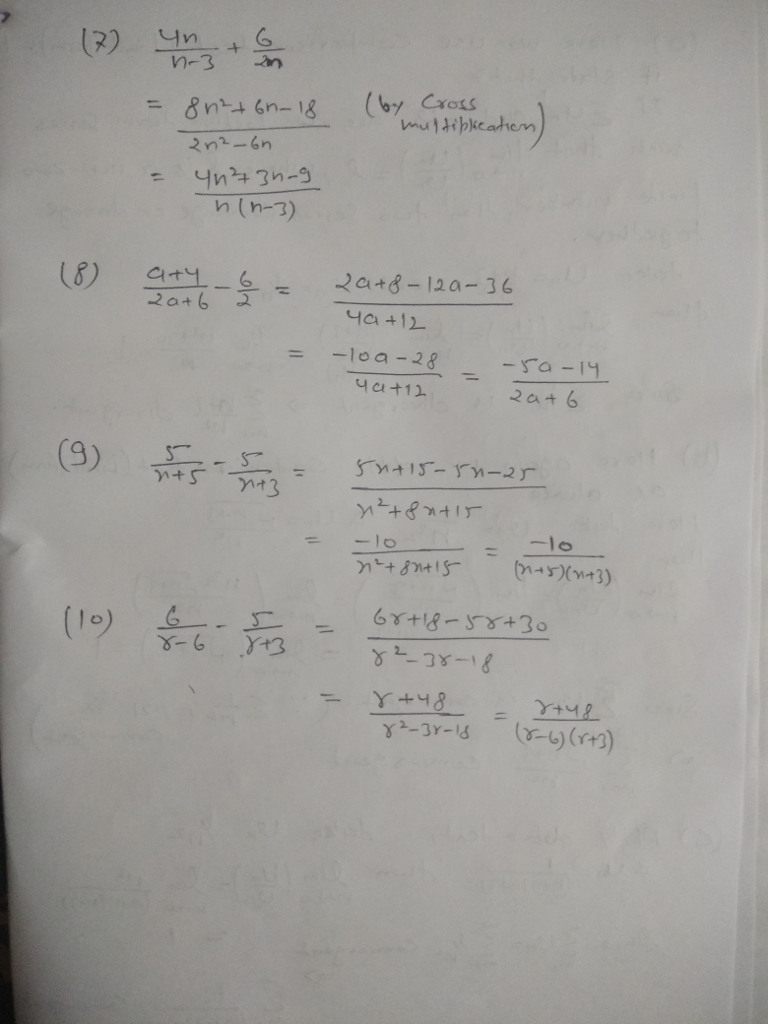#### Earn Coins

Coins can be redeemed for fabulous gifts.

Similar Homework Help Questions
• ### 1. Simplify each expression, for n = 5 a) 8n + 2 b) n2 - 2n+n...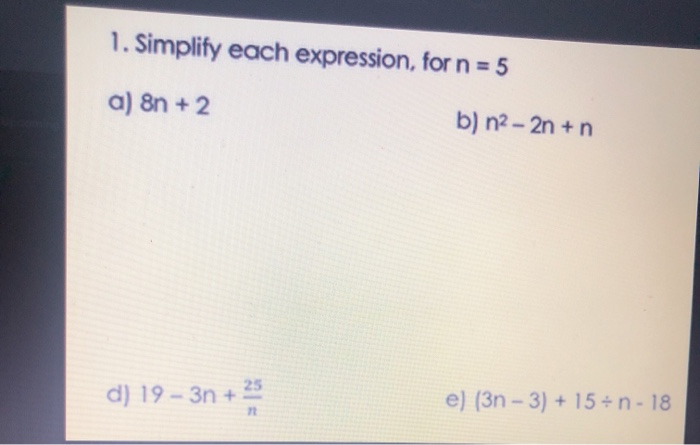1. Simplify each expression, for n = 5 a) 8n + 2 b) n2 - 2n+n d) 19 - 3n + e) (3n - 3) + 15-n-18

• ### Lim n approaches infinity# 6/n((2n)/3 + (5n(n+1))/(2n) - (4n(n+1)(2n+1))/(6n^2))=#?

lim n approaches infinity [6/n(2n/3 + 5n(n+1)/2n - 4n(n+1)(2n+1)/6n^2)]=?

• ### Use the definition of 0 to show that 5n^5 +4n^4 + 3n^3 + 2n^2 + n...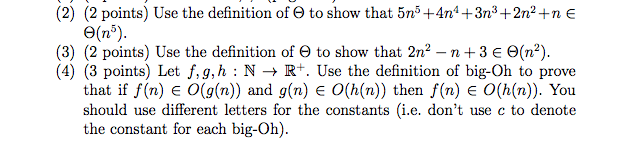Use the definition of 0 to show that 5n^5 +4n^4 + 3n^3 + 2n^2 + n 0(n^5).Use the definition of 0 to show that 2n^2 - n+ 3 0(n^2).Let f,g,h : N 1R*. Use the definition of big-Oh to prove that if/(n) 6 0(g{n)) and g(n) 0(h{n)) then/(n) 0(/i(n)). You should use different letters for the constants (i.e. don't use c to denote the constant for each big-Oh).

• ### 3 л л 4 2 6 5 5 6 1 2n n 7 12 8 and/or...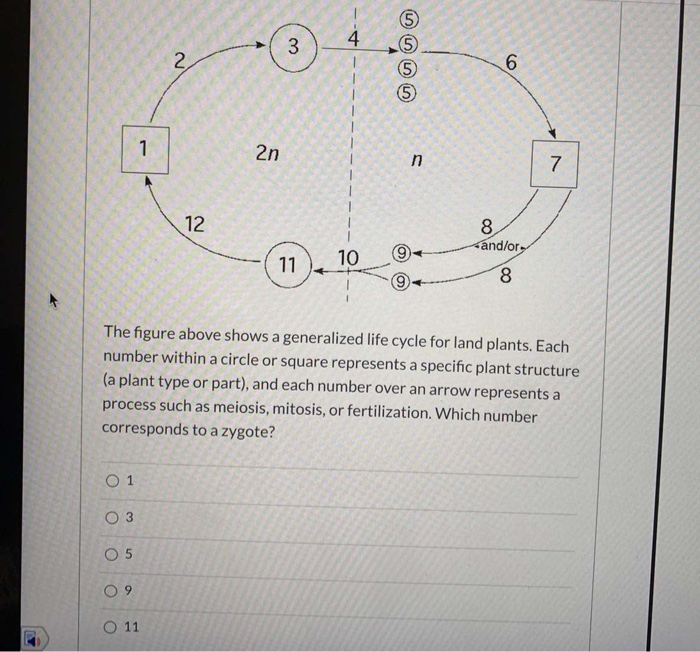3 л л 4 2 6 5 5 6 1 2n n 7 12 8 and/or 11 10 + 8 The figure above shows a generalized life cycle for land plants. Each number within a circle or square represents a specific plant structure (a plant type or part), and each number over an arrow represents a process such as meiosis, mitosis, or fertilization. Which number corresponds to a zygote? O 1 O 3 O 5 09 O 11

• ### 8 and 9 plz Multiply or divide. Simplify. (4 points each) 5x x+x-6 3x 5x+5 6)...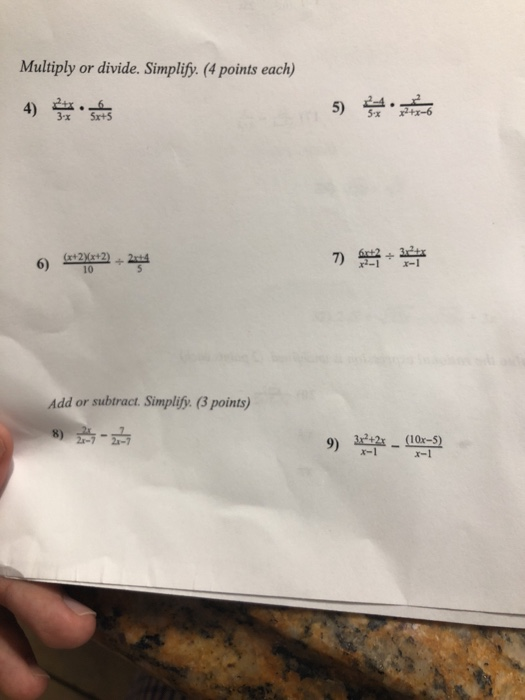8 and 9 plz Multiply or divide. Simplify. (4 points each) 5x x+x-6 3x 5x+5 6) 0 Add or subtract. Simplify. (3 points) -? 9 120S 21-7 2-7 x-1 x-1

• ### 2. Simplify: (n + 2)! (1) n! (2n-1)! (2) (2n + 1)! (2n + 2)! (3)...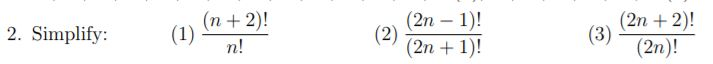2. Simplify: (n + 2)! (1) n! (2n-1)! (2) (2n + 1)! (2n + 2)! (3) (2n)!

• ### Number 3 +4n + 8 is O(n). 3. Give a formal proof that f(n) 5m3 +3n2...Number 3 +4n + 8 is O(n). 3. Give a formal proof that f(n) 5m3 +3n2 4. Give a formal proof that f(n)-7*2n+ 9m3 is O(2n). 5. Give a formal proof that log (n + 1) is O(log n).

• ### 10. Simplify each expression and write it without using factorial notation. (3 marks each) (n+4)! (n+2)!...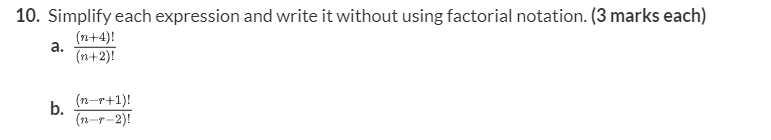10. Simplify each expression and write it without using factorial notation. (3 marks each) (n+4)! (n+2)! a. b. (n-r+1)! (n-y-2)!

• ### A tetraploid cell (4n = 8): a. could be an autotetraploid from two parents, each 2n...

A tetraploid cell (4n = 8): a. could be an autotetraploid from two parents, each 2n = 4 b. could be an allotetraploid from two parents, each 2n = 2 c. could be an allotetraploid from two parents, 1n = 2, 3n = 6 d. A or B e. A or C

• ### 9+ 5 8 7 4 h(x) 6 3 y-values 5+ y-values 4 N 8(x) 3 2...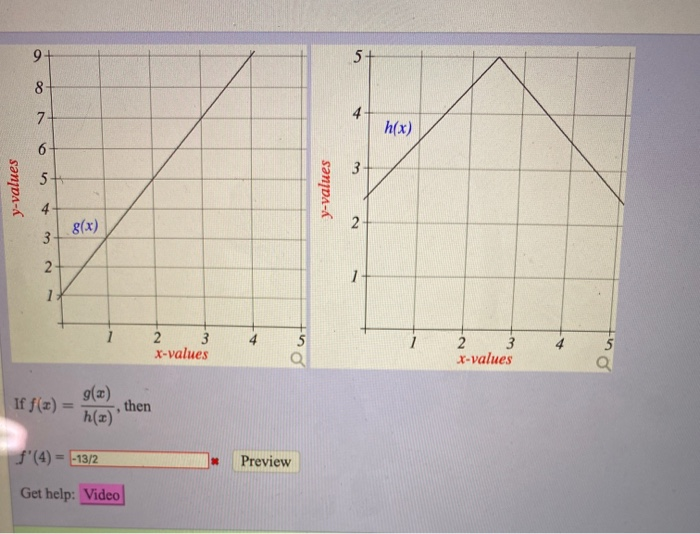9+ 5 8 7 4 h(x) 6 3 y-values 5+ y-values 4 N 8(x) 3 2 1 3 2 X-values Ou 2 3 x-values If f(x) g() then h(x)' f'(4) = -13/2 Preview Get help: Video